# Tables and Plots Flashcards

Tables and Plots Flashcards
1/17 (missed) 0 0
Create Your Account To Continue Studying

As a member, you'll also get unlimited access to over 79,000 lessons in math, English, science, history, and more. Plus, get practice tests, quizzes, and personalized coaching to help you succeed.

Try it risk-free for 30 days. Cancel anytime
Bar Graph

The amounts (frequency) of different data groups shown on a graph. This type of plot is very similar to a histogram, except that the horizontal axis is not necessarily numerical.

Got it
Stem and Leaf Display

One part of the value is shown on the left side of a display while another part is shown on the right. This type of display shows the actual data while also plotting the distribution of the data.

Got it
Skewed Distribution

The peak in a graph is not centered, but is off to the left or right. Data on the side without the peak has fewer observations, and therefore lower values.

Got it
Histogram

A graphical representation of data very similar to a bar graph showing how many times certain numbers appear in the data. ''How many times'' is called the ''frequency.''

Got it
Median

The value in the middle of an ordered set of numbers. Half of the numbers are above this value and half are below.

Got it
Interquartile Range

A measure of how the data is distributed; it's the value you get when subtracting the lower quartile value from

the upper quartile value.

Got it
Quartile

A block showing the cut-off points for a grouping of four sets of numbers in the data.

Got it
Box Plot

A representation of data on a number line showing quartiles, a minimum value, a maximum value, extreme values and a range of values.

Got it

or choose a specific lesson: See all lessons in this chapter
17 cards in set

## Flashcard Content Overview

These flashcards review important concepts related to tables and plots. These include the definitions for:

• quartile
• interquartile range
• median and
• skewed data

The types of plot you will review in these flashcards include:

• Box Plots
• Histograms
• Bar Charts
• Pie Charts
• Stem and Leaf Displays
Front
Back
Box Plot

A representation of data on a number line showing quartiles, a minimum value, a maximum value, extreme values and a range of values.

Quartile

A block showing the cut-off points for a grouping of four sets of numbers in the data.

Interquartile Range

A measure of how the data is distributed; it's the value you get when subtracting the lower quartile value from

the upper quartile value.

Median

The value in the middle of an ordered set of numbers. Half of the numbers are above this value and half are below.

Histogram

A graphical representation of data very similar to a bar graph showing how many times certain numbers appear in the data. ''How many times'' is called the ''frequency.''

Skewed Distribution

The peak in a graph is not centered, but is off to the left or right. Data on the side without the peak has fewer observations, and therefore lower values.

Stem and Leaf Display

One part of the value is shown on the left side of a display while another part is shown on the right. This type of display shows the actual data while also plotting the distribution of the data.

Bar Graph

The amounts (frequency) of different data groups shown on a graph. This type of plot is very similar to a histogram, except that the horizontal axis is not necessarily numerical.

Pie Chart

A graph of data on a circle where each sector of the circle represents a proportion of the data. The proportion is often expressed as a percentage of the total.

Calculate the number of vanilla sales; 1000 people represented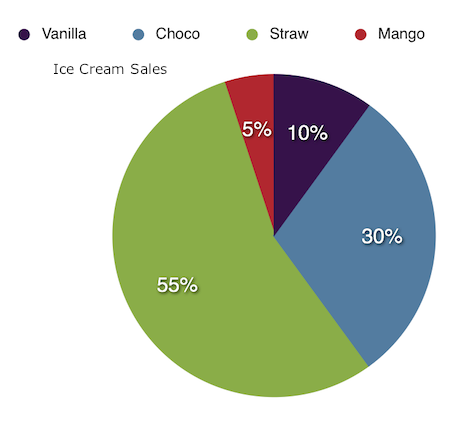100. (Vanilla represents 10% of sales. 1000 times 10% = 1000 times 0.1 = 100.)

Chocolate sales in winter?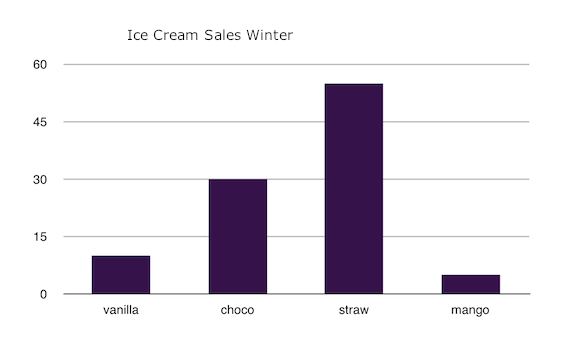30

How many 11s in the data?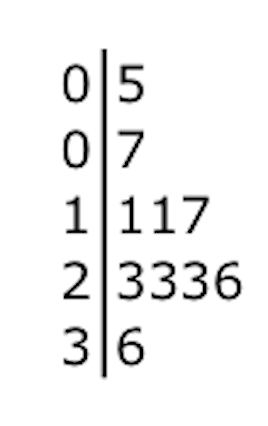2

Those in attendance for 20-30 wins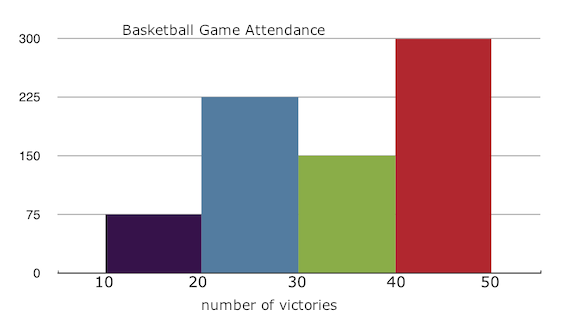225

Find the median.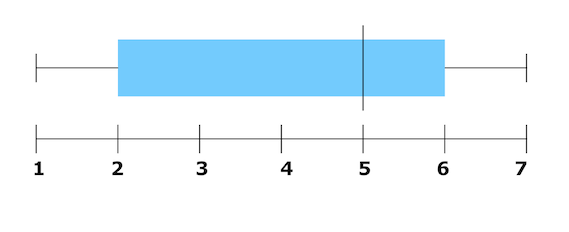5

Find the extreme maximum value.7

Bar Graph: Uses

You can use this type of graph to assess the relative frequency of how often things happen, how many people participate in a certain thing, or a value range.

Bar Graph: Bars

These represent amounts in this kind of graph. They are generally taller or longer for a larger number of items.

To unlock this flashcard set you must be a Study.com Member.